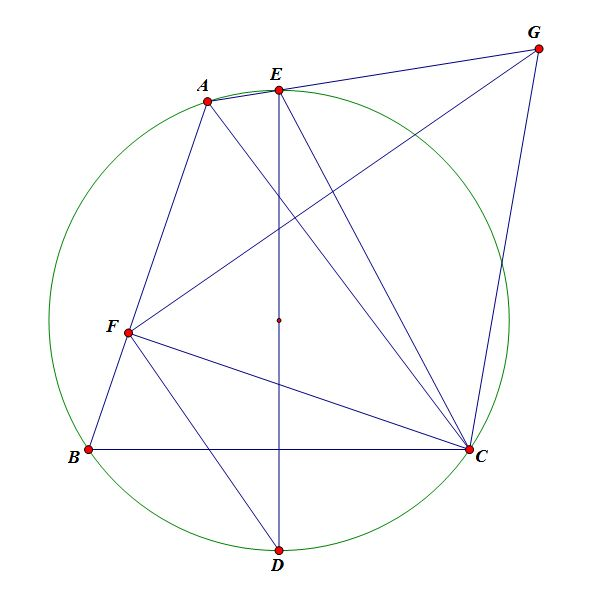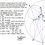# [Solutions Posted]Geometry Proof Problem of the day #3 (Diagram Added)

To get a better idea of the community's current geometric proof "skill level," I will keep dropping the difficulty of these problems until we find an adequate starting point for the synthetic geometry group. I will appreciate any feedback on today's problem as you approach it.Given $\triangle ABC$, let $D,E$ denote the midpoints of the arc $BC$, where $A,E$ lie on the same side $BC$. Construct $F$ on $AB$ such that $CF\perp AB$, and $G$ on $AE$ such that $GF\perp DF$. Prove that $CG=EC$.Note by Xuming Liang
5 years, 10 months ago

This discussion board is a place to discuss our Daily Challenges and the math and science related to those challenges. Explanations are more than just a solution — they should explain the steps and thinking strategies that you used to obtain the solution. Comments should further the discussion of math and science.

When posting on Brilliant:

• Use the emojis to react to an explanation, whether you're congratulating a job well done , or just really confused .
• Ask specific questions about the challenge or the steps in somebody's explanation. Well-posed questions can add a lot to the discussion, but posting "I don't understand!" doesn't help anyone.
• Try to contribute something new to the discussion, whether it is an extension, generalization or other idea related to the challenge.

MarkdownAppears as
*italics* or _italics_ italics
**bold** or __bold__ bold
- bulleted- list
• bulleted
• list
1. numbered2. list
1. numbered
2. list
Note: you must add a full line of space before and after lists for them to show up correctly
paragraph 1paragraph 2

paragraph 1

paragraph 2

[example link](https://brilliant.org)example link
> This is a quote
This is a quote
    # I indented these lines
# 4 spaces, and now they show
# up as a code block.

print "hello world"
# I indented these lines
# 4 spaces, and now they show
# up as a code block.

print "hello world"
MathAppears as
Remember to wrap math in $$ ... $$ or $ ... $ to ensure proper formatting.
2 \times 3 $2 \times 3$
2^{34} $2^{34}$
a_{i-1} $a_{i-1}$
\frac{2}{3} $\frac{2}{3}$
\sqrt{2} $\sqrt{2}$
\sum_{i=1}^3 $\sum_{i=1}^3$
\sin \theta $\sin \theta$
\boxed{123} $\boxed{123}$

Sort by:

Hey I got around more than half way to the problem. It's almost completed. Feeling tired. I will post it tommorow. (Feeling very excited but Tired). :P :P

- 5 years, 10 months agoimage

Hurray!!! I got the solution.

Since $EABC$ is cyclic. It implies that $\angle ABC = 180^{0} - \angle AEC = \angle CEG$.

We have to prove that $CE = EG$ i.e. $\angle CEG = \angle CGE$. It suffices to prove that $\angle CGE = \angle ABD$.

Let '$I$' be foot of perpendicular from '$C$' onto $EG$. But, $\angle EFC = \angle EIC = 90^{0}$. So, $\Delta BFC \sim \Delta CEI$. So, $\dfrac{BF}{BC} = \dfrac{EI}{EC}$ (#)

Observe that $\angle DBF = 180^{0} - \angle DEA = \angle DEG$ (1)

Since $ED$ is diameter of the circle. It implies that $\angle DAE = 90^{0}$. But $\angle DFG = 90^{0} = \angle DAG$. So, $A, G, D, F$ are cyclic.

It implies that $\angle EGD = 180^{0} - \angle AFD = \angle BFD$ (2)

From (1) and (2) it implies that $\Delta BFD \sim \Delta EGD$. So, $\dfrac{BF}{BD} = \dfrac{EG}{ED}$(##)

Dividing (#) by (##), we get,

$\dfrac{BD}{BC} = \dfrac{EI}{EC} \times \dfrac{ED}{EG}$ $\dfrac{EI}{EG} = \dfrac{BD}{BC} \times \dfrac{EC}{ED} = \dfrac{1}{2} \times \dfrac{BD}{BN} \times \dfrac{EC}{ED}$

Since, $\Delta ECD$ is right angled at '$C$' and also $\angle CED = \angle CBD$. So, $\cos{\angle CED} = \cos{\angle CBD}$. It implies that $\dfrac{EC}{ED} = \dfrac{BN}{BD}$

It gives out that $\dfrac{EI}{EG} = \dfrac{1}{2}$ i.e. $EG = 2 EI$. So, $EI =GI$. It implies that $\angle CEG = \angle CGE$ i.e. $CG = CE$ as required.

SPECIAL THANKS TO @Xuming Liang

- 5 years, 10 months ago

Nice solution! Well Done. I have understood your solution and I realize that I was close to prove it. But I think there are few trivial typo mistakes at start that you should fix. Overall your solution is quite rich! :) :) :)

- 5 years, 10 months ago

Congradulations :) You are the first Brilliant solver of my challenges. Great Job

- 5 years, 10 months ago

A slightly alternate solution:

Since $DA\perp AG, DF\perp GF$, $D,F,A,G$ are concyclic and thus $\angle DGA=\angle DFB$. In addition,note that $\angle DBA=\angle DEG$, therefore $DEG\sim DBF$. At this point one can use the new-found information to ratio chase $GE$ related lengths, as Surya Prakash has demonstrated, or we can continue to utilize similar (they are almost the same thing),

Since $DEG\sim DBF$, we ask what point corresponds to $C$ in the $DBF$ configuration, i.e. For what point is it to $DBF$ as $C$ is to $DEG$(Not sure if this makes sense...) By some thought we quickly see that it is the midpoint of $BC$ denoted by $M$ (I will elaberate: it must lie on $BC$ since $\angle GEC=\angle FBC$, and $M$ is the only point that satisfy $BM=FM$). Clearly $DBM\sim DEC$, thus $DEGC\sim DBFM$. SInce $BM=FM$, we have $CG=EC$ and are done.

Similarity is powerful isn't it?

- 5 years, 10 months ago

Nice and easy one.

- 5 years, 10 months ago

Can you post the figure?

- 5 years, 10 months ago

01000011 01101111 01101110 01100111 01110010 01100001 01110100 01110011 00100000 01101111 01101110 00100000 00110001 00110000 00110000 00110000 00100000 01000110 01101111 01101100 01101111 01101111 01110111 01100101 01110010 01110011 00100001

- 5 years, 10 months ago

What's that @Mehul Arora

- 5 years, 10 months ago

I'm sure Agnishom will understand :)

- 5 years, 10 months ago

Tell me too :P @Mehul Arora

- 5 years, 10 months ago

Come to Slack ;)

- 5 years, 10 months ago

74 68 61 6e 6b 20 79 6f 75 21

- 5 years, 10 months ago

Binary

- 5 years, 10 months ago

Yeo. Binary it is :P

- 5 years, 10 months ago

I guess this is a geometrical discussion forum... Or wait , are you thinking of computer scientific geometry? :O :3

- 5 years, 10 months ago

Yep. Computer scientific geom would be better :P

- 5 years, 10 months ago

You mean computational geometry?

- 5 years, 10 months ago

Yeah.

Leave it , I was just joking :P

- 5 years, 10 months ago

$\large{27741264475741985937324611021968519394650689232351903626759926561}$

- 5 years, 10 months ago

What difficulty are you encountering in drawing the diagram? May be I can save your time by helping you out :)

- 5 years, 10 months ago

Can you help me understand how I construct the arc?

- 5 years, 10 months ago

Draw circumcircle of $\Delta ABC$. Now draw perpendicular bisector of $BC$ and let it intersect the circumcircle at $D,E$ and we have $D,E$ as mid-points of arc $BC$. Now suppose if $A$ lies on major arc $BC$ , then $E$ must also lie on major arc and same if its case of minor arc.

- 5 years, 10 months ago

Did u get the problem @Nihar Mahajan

- 5 years, 10 months ago

Ah ,Don't expect me to solve Xuming's challenge so early :P I am not as awesome as he is ;)

- 5 years, 10 months ago

Also , Congrats for having 1000 followers! :P :) :3

- 5 years, 10 months ago

Thanks! I decided that I will be excited at first, but then again, I said, I thought I will save the excitement for 1024 followers instead

- 5 years, 10 months ago

Why 1024 only? Wait , you planning something awesome?

- 5 years, 10 months ago

So basically, we have to prove that G and E coincide, right?

- 5 years, 10 months ago

No. Not at all. In short you must prove that triangle CGE is isosceles.

- 5 years, 10 months ago

@Xuming Liang Do D and E lie on the circumcirlce of $\Delta ABC$?

- 5 years, 10 months ago

Yes , they do.

- 5 years, 10 months ago